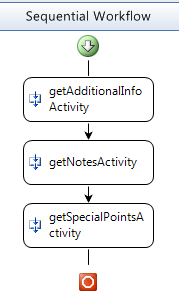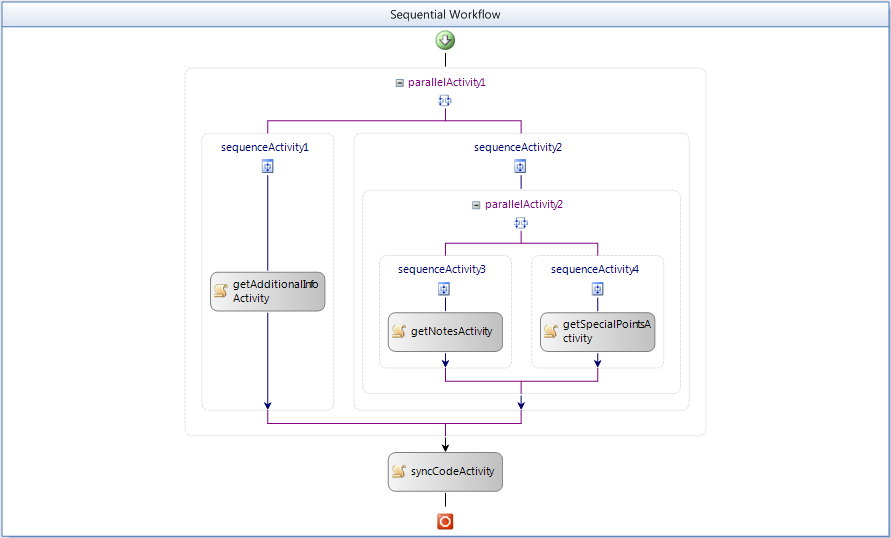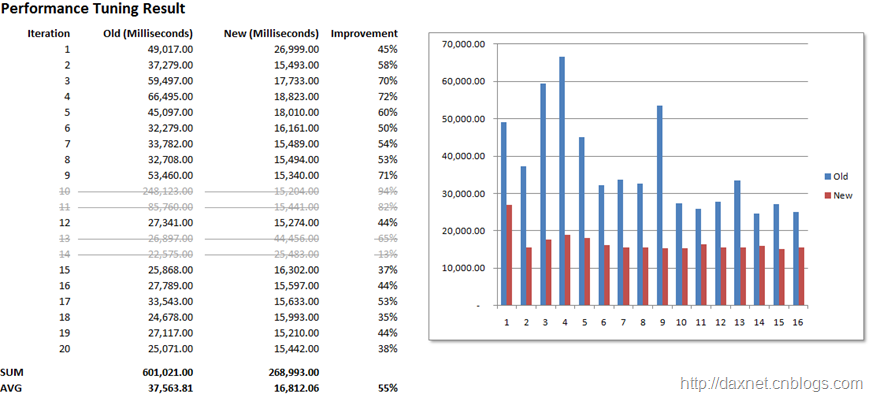# 在.NET Workflow 3.5中使用多线程提高工作流性能• 三个Activity的输入属性参数都来自于Workflow（即通过与Workflow中定义的DependencyProperty进行绑定而获得数据），并不存在下游的Activity的输入参数需要依赖上游Activity的输出参数的情况
• 每个Activity的输出属性参数都只关注某一种类型的数据，在Workflow Runtime执行完某个Activity后，也会通过DependencyProperty将处理结果传递给Workflow，而这些输出属性参数之间也并没有任何关联
• 三个Activity所调用的Data Service也比较独立，基本上可以说是在做三个完全不同的工作
• 时间主要消耗在Data Service的调用上，不存在由于复杂的运算逻辑导致CPU利用率近似100%的情况，也不存在由于物理内存用完而需要频繁读写虚拟内存的情形1. ```using System;
```
2. ```using System.Collections.Generic;
```
3. ```using System.Threading;
```
4. ```using System.Workflow.Activities;
```
5.

6. ```namespace WorkflowConsoleApplication3
```
7. ```{
```
8. ```    public sealed partial class Workflow1 : SequentialWorkflowActivity
```
9. ```    {
```
10. ```        List<Thread> threads = new List<Thread>();
```
11.

12. ```        public Workflow1()
```
13. ```        {
```
14. ```            InitializeComponent();
```
15. ```        }
```
16.

17. ```        private void getAdditionalInfoActivity_Execute(object sender, EventArgs e)
```
18. ```        {
```
19. ```            var t1 = new Thread(() =>
```
20. ```                {
```
21. ```                    // Call Data Service 1 to implement business logic...
```
22. ```                });
```
23. ```            threads.Add(t1);
```
24. ```            t1.Start();
```
25. ```        }
```
26.

27. ```        private void getNotesActivity_Execute(object sender, EventArgs e)
```
28. ```        {
```
29. ```            var t2 = new Thread(() =>
```
30. ```                {
```
31. ```                    // Call Data Service 2 in a loop to implement business
```
32. ```                    // logic...
```
33. ```                });
```
34. ```            threads.Add(t2);
```
35. ```            t2.Start();
```
36. ```        }
```
37.

38. ```        private void getSpecialPointsActivity_Execute(object sender, EventArgs e)
```
39. ```        {
```
40. ```            var t3 = new Thread(() =>
```
41. ```                {
```
42. ```                    // Call Data Service 3 to implement business logic...
```
43. ```                });
```
44. ```            threads.Add(t3);
```
45. ```            t3.Start();
```
46. ```        }
```
47.

48. ```        private void syncCodeActivity_Execute(object sender, EventArgs e)
```
49. ```        {
```
50. ```            // Wait for all threads to terminate...
```
51. ```            threads.ForEach(p => p.Join());
```
52.

53. ```            // TODO: Process with results and exceptions
```
54. ```        }
```
55. ```    }
```
56.

57. ```}
```
58.

59.